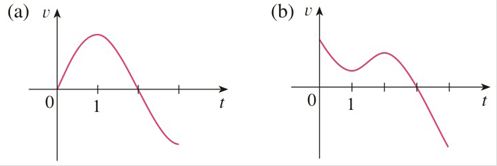Chapter 2.7, Problem 5E

Chapter
Section
Textbook Problem

# Graphs of the velocity functions of two particles are shown, where t is measured in seconds. When is each particle speeding up? When is it slowing down? Explain.To determine

Part (a)

To explain: When is each particle speeding up and speeding down

Explanation

Acceleration is rate of change of velocity with respect to time that is acceleration is the derivative of velocity of the particle.

From the above graph velocity is positive on the interval (0, 2) and is negative on the interval (2, 3).

Acceleration is positive on the interval (0, 1) since slope of the graph is positive.

And negative on the interval (1, 3) since slope of the graph is negative

To determine

Part (b)

To explain: When is each particle speeding up and speeding down

### Still sussing out bartleby?

Check out a sample textbook solution.

See a sample solution

#### The Solution to Your Study Problems

Bartleby provides explanations to thousands of textbook problems written by our experts, many with advanced degrees!

Get Started

#### Convert the expressions in Exercises 8596 radical form. x4/3

Finite Mathematics and Applied Calculus (MindTap Course List)

#### 32. If , find the following. (a) (b) (c) (d)

Mathematical Applications for the Management, Life, and Social Sciences

#### Convert the following percents to decimals. 422%

Contemporary Mathematics for Business & Consumers